### Find the Nth Fibonacci Number – C# Code

The Fibonacci sequence begins with Fibonacci(0) = 0  and Fibonacci(1)=1  as its respective first and second terms. After these first two elements, each subsequent element is equal to the sum of the previous two elements.

Here is the basic information you need to calculate Fibonacci(n):

• Fibonacci(0) = 0
• Fibonacci(1) = 1
• Fibonacci(n) = Fibonacci(n-1) + Fibonacci(n-2)

Given n, complete the fibonacci function so it returns Fibonacci(n).

Input Format

Nth number

Output Format

Fibonacci() Nth number.

Sample Input

Sample Output

Explanation

Consider the Fibonacci sequence: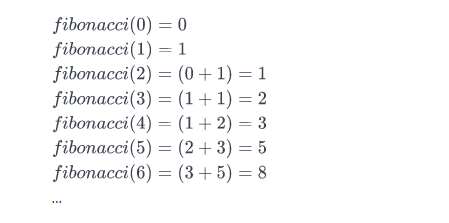We want to know the value of Fibonacci(3). If we look at the sequence above,Fibonacci(3)  evaluates to 2. Thus, we print 2 as our answer.

### Array Left Rotation by D – C# Code

left rotation operation on an array of size n shifts each of the array’s elements 1 unit to the left. For example, if 2 left rotations are performed on array [1,2,3,4,5], then the array would become  [3,4,5,1,2].

Given an array of  n integers and a number, d , perform d left rotations on the array. Then print the updated array as a single line of space-separated integers.

Input Format

The first line contains two space-separated integers denoting the respective values of  (the number of integers) and  (the number of left rotations you must perform).
The second line contains  space-separated integers describing the respective elements of the array’s initial state.

Output Format

Print a single line of  space-separated integers denoting the final state of the array after performing  left rotations.

Sample Input

Sample Output

Explanation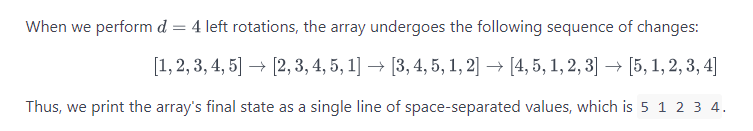Code

We can see the results for 1 and 4 rotations as follows:### Bottom Navigation Bar with Control – Xamarin.Forms

Ever wondered how to create a Bottom Navigation Bar for Xamarin.Forms as it is in the latest Android sdk version? As there is no native control for Xamarin.Forms you could create a custom one with a Control.

First create a Folder and name it Controls and create the control in there.

In your portable class library -> Add New Item -> Content View and name it NavigationBar or something else.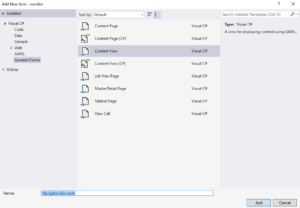For my purposes I used the following code:

In order to use it in your code reference it in your xaml

And then just use

Finally you can use your control and it will look the same in all platforms.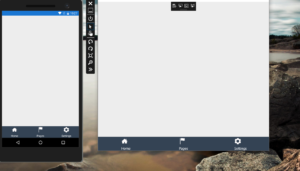### Local Database with Realm – Xamarin.Forms

Realm is a very popular solution for mobile databases as it includes very nice features, it’s very quick and easy to use. It supports many platforms as well as Xamarin.

You can use realm with Xamarin.Forms after installing the nuget package. Be careful to install nuget package to Android, iOS and UWP seperate project.Current realm version is 1.5. After choosing Realm package, nuget will also install all available requirements.

First of all in order to store items in the database, you should create a model which will represent the identity that you want to save. For example lets say that we want a Person identity so we will store Persons.

Lets create the necessary model for this case:

After that we  will create a test interface in which we can add some users and interact with realm.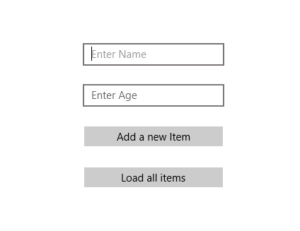To interact with the database lets create a RealHelper class which we will use to add persons and load.

In order to make a write operation we use a transaction and we do that by creating a write with beginWrite(). More information for the functions and how they operation can be found on the documentation.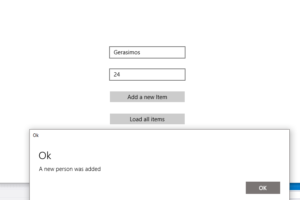The main logic comes from the two buttons:

Putting a breakpoint in the loadPersons button we can see the stored persons.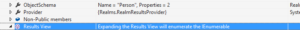initialization issues I found:

• In the UWP project you must install realm database nuget package and not realm nuget package. That’s because some functions don’t exist in the uwp apis so if you install realm package some exceptions will occur during the opening of the app.
• Check if fody file exists in both iOS, Android and UWP projects and the its content should be

• Be sure that all packages are updated across all projects and are the same version.